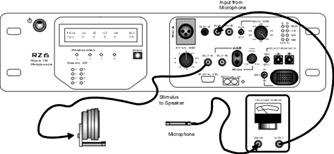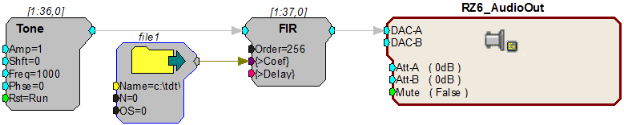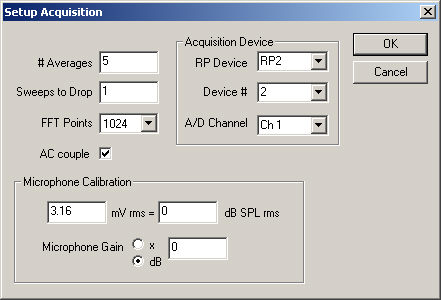# SigCalRP Tech Notes

Normalize speaker output, using an FIR filter in an RPvdsEx circuit created in Matlab

## TN0966

Product: RPvdsEx, SigCalRP
Version: All

### Issue

This article explains how to use SigCalRP and Matlab to generate FIR coefficients, then use them in RPvdsEx to filter signals that will be played out to a speaker.

1. Use SigCalRP to measure the transfer function (TF) of the system.

2. Export calibration data in CSV format.

3. Use a Matlab script to convert the CSV file into a set of filter coefficients.

The FIR coefficients generated in this example, can be used to filter signals of varying frequency and constant amplitude so that the sound output in units of dB SPL from the speaker will be constant over all frequencies. These coefficients will be generated for a circuit running at a specific sampling frequency. To work properly, they must be used in a circuit running at the same sampling frequency.

Measuring the TF of the system

SigCalRP can be used to calculate the TF of a system for speaker calibration at sample rates up to 200 kHz. Before performing this operation, users should know the A/D and D/A conversion limitations of their specific hardware. For example, the RZ6 maximum sampling rate is 195312.50 for both signal production and acquisition. The figure below shows a typical setup using a single RZ6.Under Signal Setup in SigCalRP, enter the frequency step using the number of frequencies you wish to measure across the spectrum and the Nyquist frequency. For an RZ6 running at 200 KHz and a filter of 200 points, the Nyquist frequency is 97656.25 Hz and the frequency start and frequency step are determined by the relationship:

97656.25/200 = 488.28125 Hz

Run SigCalRP at 200 kHz to ensure that the Nyquist frequency of the device running the FIR (97656.25 Hz) is represented. Also, make sure that all test frequencies used are integer multiples of the Nyquist frequency of the device to avoid aliasing.

Set the horizontal green bar in the calibration plot to the dB level you want to achieve for all frequencies using the filter. The filter will attenuate frequencies above the green bar and amplify frequencies below it. Use care when amplifying the signal. Typically, only frequencies that will not be presented should be amplified.

To export the data as a *.csv file, select Export Calibration Data on the Calibrate menu.

Creating the filter coefficients

Next, download and extract the compressed (.zip) SigCalRP Example files from the TDT website at SigCalRPExamples.zip.

Use the provided Matlab script (RZ6SigCalFIR.m) to convert the CSV file into a set of FIR coefficients. Your output will be a .txt file that can be used with RPvdsEx.

Add FIR and SourceFile components to the signal generation segment of your RPvdsEx circuit as shown below.Update the SourceFile component to point to the .txt file generated by the Matlab script. Any signal that passes through that FIR component will be normalized.

A sample CSV file and several circuit files are included in the Example folder for demonstration purposes.

Frequency End value is not being included for multiplicative step

## TN0851

Product: SigCalRP
Version: 4.2

### Issue

When performing a multiplicative step in SigCalRP, the designated Frequency End value is not included.

For example, if Frequency Start = 1000, Frequency End = 16000, Frequency Step is 2 the series will yield:

1000, 2000, 4000, 8000.

### Workaround

Add 1 to the desired value you wish to use for Frequency End.

For example, if Frequency Start = 1000, Frequency End = 16001, Frequency Step is 2, the series will then yield:

1000, 2000, 4000, 8000, 16000.

### Solution

Calibration of a hydrophone using SigCalRP

## TN0828

Product: SigCalRP
Version: All

### Issue

Hydrophones and underwater calibration equipment use different calibration values than the calibration in microphones. SigCalRP is configured for measuring a signal in SPL (Sound Pressure Level). An SPL of 0 dB is the hearing threshold of a normal human at 1000 Hz. Calibration of a signal in water requires modification to the terms used in SigCalRP in order to match the calibration terminology of a hydrophone.

### Solution

The following example illustrates the conversion process for hydrophone calibration in SigCalRP:

The RESON TC4032 Hydrophone is a typical hydrophone used for underwater sound pressure measurements. Often, sound pressure measurements in water are measured in reference to micro pascal's (uPa. The hydrophone sensitivity is -170 dB re 1V / uPa. This means that a micro pascal pressure wave will generate a voltage of -170 dBV on the hydrophone. We must first convert the units of reference, in this case micro pascal of pressure to pascal. This is done by scaling the sensitivity by a factor of 1 million (1 Pa = 1,000,000 uPa). To do this we scale the voltage reference by the equivalent value in dB (1,000,000 times increase = 120 dB).

-170 dB + 120 dB = -50 dB re 1V / Pa

SigCalRP calibration specifies milliVolts rms to dB SPL rms. In order to calibrate the hydrophone, we need a measurement of mV rms for the base sensitivity of the hydrophone. This is accomplished by converting the hydrophone's sensitivity from dB to its corresponding voltage.

To do this we use a voltage ratio conversion: 10 ^( - 50 dBV / 20 ) = 3.16 mV

Finally, to calibrate SigCalRP we set the value of our converted sensitivity to 0 dB SPL. This means that 3.16 mV will correspond to 0 dB SPL. For reference, we may equate this 0 dB SPL to 1 Pa of pressure when viewing SigCalRPs output. Therefore, any measurements shown in SigCalRP will calibrate to 3.16 mV = 0 dB SPL or 1 Pa of pressure.

To set the calibration in SigCal:

1. Select Acquisition from the Setup menu to prompt the Setup Acquisition dialog box.

2. In the Microphone Calibration area enter 3.16 mV rms for the signal voltage value and 0 dB SPL rms for the pressure reference.3. Click OK.

SigCalRP is now successfully calibrated to the RESON TC4032 hydrophone.

Note

It is also important to adjust the gain of the hydrophone to match the A/D converters on the acquisition device.

'Failed to save document' error

## TN0824

Product: SigCalRP
Version: 4.2 version

### Issue

SigCalRP will be unable to save a *.cal file even with less than 100 frequencies specified.

### Solution

SigCalRP crashes when saving .cal file

## TN0095

Product: SigCalRP
Version: All